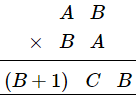# Replace A, B, C by suitable numerals.

Question:

Replace A, B, C by suitable numerals.Solution:

$A \times B=B \Rightarrow A=1$In the question:

First digit = B+1

Thus, 1 will be carried from 1+B2 and becomes (B+1) (B2 -9) B.

∴ C = B2 -1

Now, all B, B+1 and B2 -9  are one digit number.

This condition is satisfied for B=3 or B=4.

For B< 3, B2 -9 will be negative.

For B>3, B2 -9 will become a two digit number.

For B=3 , C = 32 - 9 = 9-9 = 0

For B = 4, C = 42 -9 = 16-9 = 7